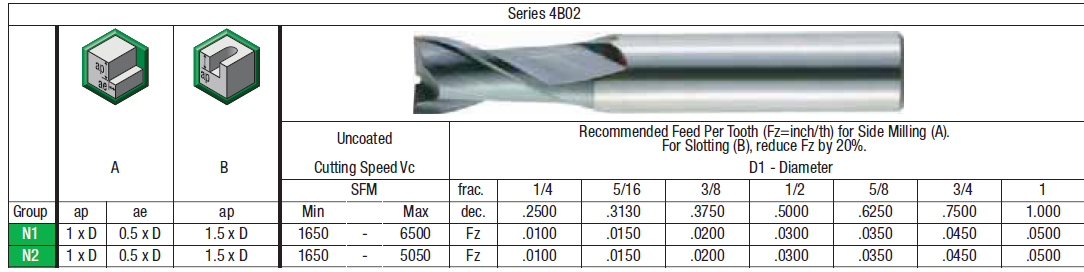Categories
Pinboard
Subscribe to Blog

## What are Machining Speeds and Feeds

One of the primary tasks machinists must learn to perform is a calculation of speeds and feeds required for milling, drilling, and turning.

It starts with knowing what workpiece material you have and what tooling and how you will be using to machine it.
The combination of these two factors determines your initial Cutting Speed and Chip Load that you can put into the speed and feed formulas to calculate the cutting tool RPM and feed rate.

Cutting Speed is the speed at which the tip of the tool travels through the material. It is commonly expressed in Surface Feet per Minute (SFM) or Surface Meters per Minute (SMM).

Chip Load is the advancement of each tooth per revolution of the tool.

In other words, Chip Load is the thickness of the material that each tooth removes per each revolution.

## So how do you find the Cutting Speed and Chip Load for your tool?

Tool manufacturers often post Cutting Speeds and Feeds for their tools for various materials and cutting conditions.

Most experienced machinists simply remember cutting speeds and chip loads for materials they machine most often.

Here are commonly recommended cutting speeds and chip loads for carbide tools for a couple of materials:

• Aluminum: 300SFM, 0.7% of the diameter (for example fz = 0.5"dia x 0.007 = 0.0035in/tooth)

• Annealed Tool steel: 150SFM, 0.4% of the diameter (for example fz = 0.5" x 0.004 = 0.002in/tooth)

When you have manufacturers' data simply find your tool in the catalog and cross-reference the cutting speed and chip load against the tool diameter:Since cutting speeds can be in either Imperial (SFM) or Metric (SMM or m/min) units, you have to use two formulas to calculate the RPM.

## Imperial Speed and Feed Calculation

Code

 RPM= 12 x SFM = Please enter Speed and Diameter 3.14 x in

Feed Rate = RPM x x in = Please enter RPM, number of teeth, and chip load (in/min)

## Metric Speed and Feed Calculation

Code

 RPM= 1000 x m/min = Please enter Speed and Diameter 3.14 x mm

Feed Rate = RPM x x mm = Please enter RPM, number of teeth and chip load (mm/min)

Want something better?

### More about formulas needed to calculate turning, drilling, and of course milling speeds and feeds

Here are a few basic formulas with term explanations to get you going:

## Calculating Spindle Speed (RPM) Using Imperial Cutting Speed:

Code

 RPM= 12 x SFM 3.14 x Diameter

Simplified imperial formula:

 RPM= 3.8 x SFM Diameter

## Calculating Spindle Speed (RPM) using Metric Cutting Speed:

Code

 RPM= 1000 x SMM 3.14 x Diameter

Simplified metric formula:

 RPM= 318 x SMM Diameter

## Calculating Feed Rate :

Feed_Rate=RPM x N(teeth) x CL(chip load)

#### Definitions

• RPM = Revolutions Per Minute, Spindle Speed, Number of revolutions of spindle per minute
• SFM = Imperial. Surface Feet Per minute, Cutting speed, Speed at which the tip of the tool travels through material
• SMM= Metric. Surface Meters Per minute, Cutting speed, Speed at which the tip of the tool travels through material
• Feed_Rate = Inches Per Minute or Millimeters Per Minute, Feed Rate, Distance in inches or millimeters the tool travels through workpiece per minute.
• N = Number of teeth on the cutter
• CL= Chip Load per tooth, This is the advancement of the cutter per revolution per cutting edge. Otherwise generally explained as the thickness of material each tooth takes per each revolution

### Examples:

Calculate Speeds and Feeds for 1/2" (0.5 in) 2 flute end mill in Mild Steel at cutting speed = 100(ft/min), Chip Load=0.001(inch per tooth)

#### Calculating Spindle Speed (RPM):

Code

 RPM= 12 x SFM 3.14 x Diameter

=

 RPM= 12 x 100(ft/min) =764(revs/min-1) 3.14 x 0.5(in)

For turning applications, we do not need this formula since Spindle Speed is usually given in Constant Surface Speed (CSS), which uses SFM value directly. But if you still want to use the RPM formula, then the diameter value is the actual diameter of the workpiece.

#### Calculating Feed Rate in Inches Per Minute (IPM)

The formula is used for milling and drilling applications. Please note that some tool manufacturers provide their recommended feed rate as feed per revolution. In such cases do not multiply by the number of teeth.

Code

Feed Rate = RPM x N x CL

Feed Rate = 800(RPM) x 2(teeth) x  0.001("chip load) =1.600 (in/min)

## Easily Calculating Speeds and Feeds on your smartphone or computer

Our own free Speed and Feed Calculator can help you calculate cutting parameters for many different material groups.

No Comments posted yet, be the first one!

# New Comment to: General Speeds and Feeds Formulas

Name: *
Solve Capcha:*
Sing In